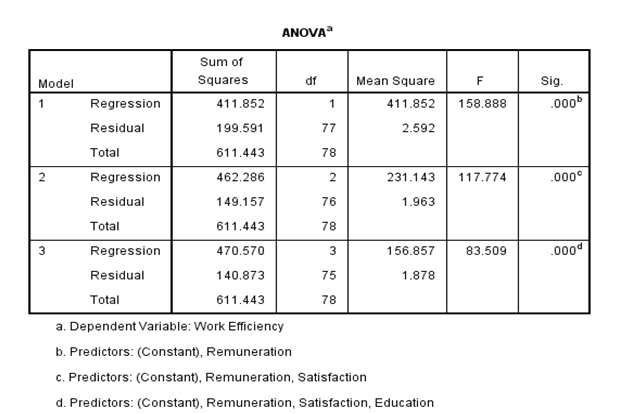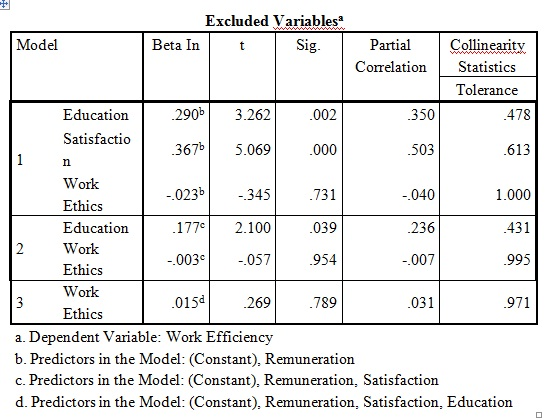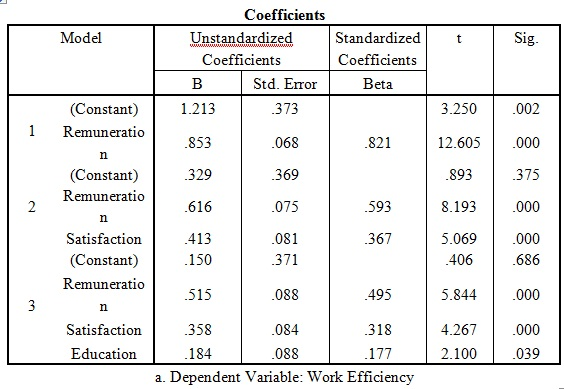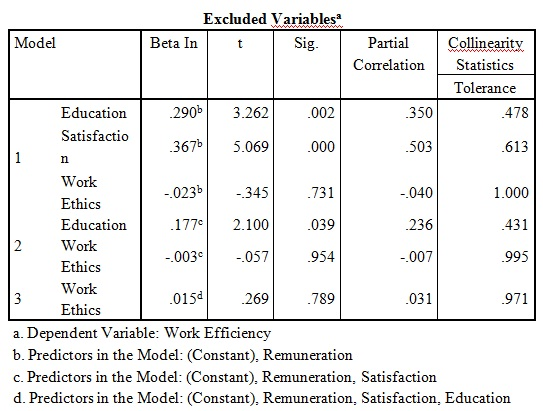# How to perform LASSO regression test?

In statistics, to increase the prediction accuracy and interpret-ability of the model, Least Absolute Shrinkage and Selection Operator (LASSO) is extremely popular. It is a regression procedure that involves selection and regularisation and was developed in 1989. LASSO regression is an extension of linear regression that uses shrinkage. The LASSO imposes a constraint on the sum of the absolute values of the model parameters. Here the sum has a specific constant as an upper bound. This constraint causes regression coefficients for some variables to shrink towards zero, i.e. ‘shrinkage’. The LASSO regression is easy when there is automatic feature or variable selection. It is also useful when dealing with predictors with high correlation, where standard regression will usually have large regression coefficients.

## How LASSO regression works?

Application of LASSO regression takes place in three popular techniques; stepwise, backward and forward technique.

1. Stepwise model begins with adding predictors in parts. Here the significance of the predictors is re-evaluated by adding one predictor at a time. That means, one has to begin with an empty model and then add predictors one by one.
2. Backward model begins with the full least squares model containing all predictors. Then it iteratively removes the least useful predictor, one-at-a-time. In order to perform backward selection, observations in the data set should be more than variables. This is because one can perform least squares regression when observations are greater than number of independent variable.
3. Forward model to choose a subset of the predictor variables for the final model. One can do forward model in context of linear regression whether observations are less than variables or the other way round. Forward selection is a very attractive approach, because it’s both tractable and it gives a good sequence of models.

## Example of LASSO regression

This section shows a practical example of how LASSO regression works. A sample data contains work-efficiency as the dependent variable and education, work ethics, satisfaction and remuneration are independent variables. Load the data set in SPSS using the following steps:

### Analyze > Regression > Linear > Stepwise method

Starting from stepwise method, table 1 below provides all the variables that show the significance p value (that is less than 0.05) in the model, the variables are remuneration, satisfaction and education.Table 1: Variables entered and removed in LASSO regression example in SPSS (Stepwise method)

The ANOVA table 2 below also shows the significant p value for all the above variables. Now, in stepwise regression at each step one variable is added, so at the final row once can see that the work ethics is not included in the model because p value (0.78) is greater than 0.05.Table 2: ANOVA test for LASSO regression example in SPSS (Stepwise method)Table 3: Excluded variables for LASSO regression test on SPSS (Stepwise method)

### Analyze > Regression > Linear > Backward

This model begins with a full model and one predictor is removed at a time. All the variables are entered into the model than the independent variable with the partial small correlation consider for the removal. So after running the backward method, Table 4 below shows the partial coefficients for the education, satisfaction and remuneration variables. The significant p value is less than 0.05 except for one variable, work ethics. Since it does not show the significant p value, remove that variable.Table 4: Coefficients for LASSO regression test on SPSS (Backward method)

### Analyze > Regression > Linear > Forward

The partial coefficients in table 5 are for variables present in the model whereas table 6 the coefficients which are absent from the model. One can see that the work ethics is absent in this case also.Table 5: Coefficients for LASSO regression test on SPSS (Forward method)Table 6: excluded variables for LASSO regression test on SPSS (Forward method)

## Applications of LASSO regression

• LASSO regression is important method for creating parsimonious models in presence of a ‘large’ number of features.
• Its techniques help to reduce the variance of estimates and hence to improve prediction in modeling.
• It helps to deal with high dimensional correlated data sets (i.e. DNA-microarray or genomic studies).
• It is also useful in high-dimensional feature selection and prediction in many bioinformatics and bio statistical contexts.
• It is popular in genomic data and two genome-scale experimental datasets.
• LASSO regression is popular in reducing dimensionality and computation time.
• LASSO methods also assess prediction accuracy in independent test data
• It is popular in the field of machine learning, computer vision, and artiﬁcial intelligence.

## Software supporting LASSO regression

There are lots of software present in statistics that supports Lasso regression applications with multiple independent variables such as R, SAS, MATLAB, STATA and SPSS.

### Prateek Sharma

Analyst at Project Guru
Prateek has completed his graduation in commerce with a rich experience in Telecom, Marketing and Banking domains for preparing comprehensive documents and reports while managing internal and external data analysis. He is an adaptable business-minded Data Analyst at Project Guru skilled in recording, interpreting and analysing data with a demonstrated ability to deliver valuable insights via data analytics and advanced data-driven methods. Apart from his strong passion towards data science, he finds extreme sports interesting. He keeps himself updated with the latest tech and always love to learn more about latest gadgets and technology.

#### Latest posts by Prateek Sharma (see all)We are looking for candidates who have completed their master's degree or Ph.D. Click here to know more about our vacancies.